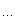1 newtype Mu a = Mu (Mu a -> a)
2
3 y :: (a -> a) -> a
4 y f = (\h -> h \$ Mu h) (\x -> f . (\(Mu g) -> g) x \$ x)

1 Y = function (f)
2    return function()
3       return (function(x) return x(x) end)
(function(x) return f(function(y) return x(x)(y) end) end)()
4    end
5 end

λf. (λx. f (x x)) (λx. f (x x))

f a \$ Mu a，这时候Mu a = Mu (Mu a -> a) 递归定义的作用就发挥了，为了类型的推导，继续将那个红色的a 推导成 Mu a -> a，这样 f (Mu a -> a) 会返回一个Mu a -> a，管他叫f'把，这样 f' (Mu a) 就返回一个 a。有根据前面的(\h -> h \$ Mu h) 继续讲上面提到的a变成 Mu a -> a。就是把Mu a 喂给了 (Mu a -> a)，最后还是返回一个a。
(>_< 其实上面这段是我编出来的，我编不下去了，我不知道ghc是怎么做这个事情的，等我有生之年看完slpj-book-1987再想想)

y (\f n -> if n <= 1 then 1 else n * f (n - 1)) 5。

posted on 2014-02-27 00:25 右席 阅读(2136) 评论(5)  编辑 收藏 引用 所属分类: 搬砖之路